# 图文实例教会你逻辑回归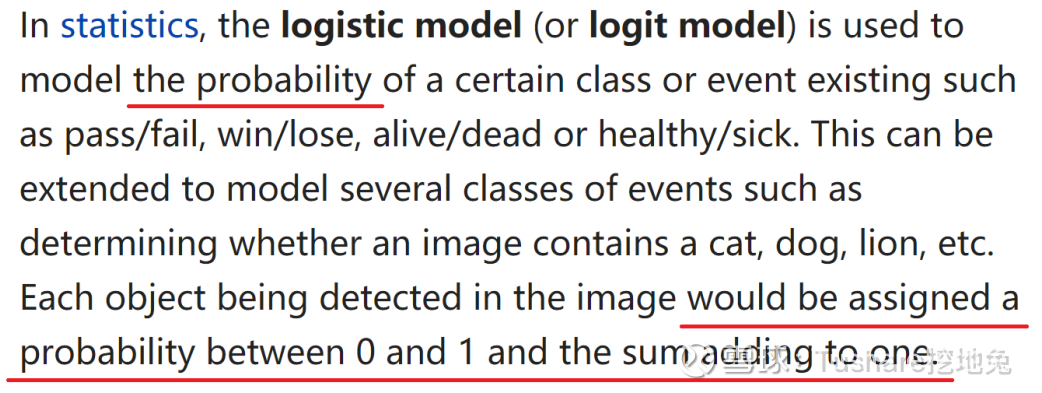① 获取数据，包含重量和尺寸。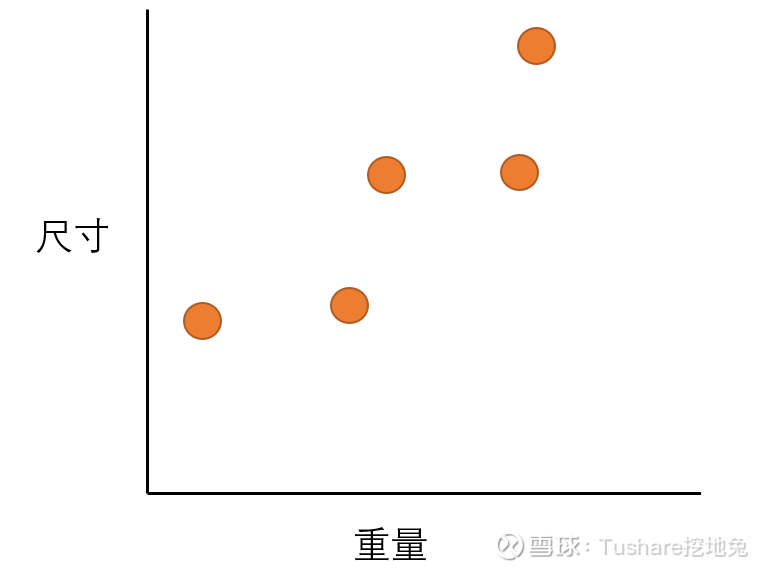② 建立模型，拟合出一条直线。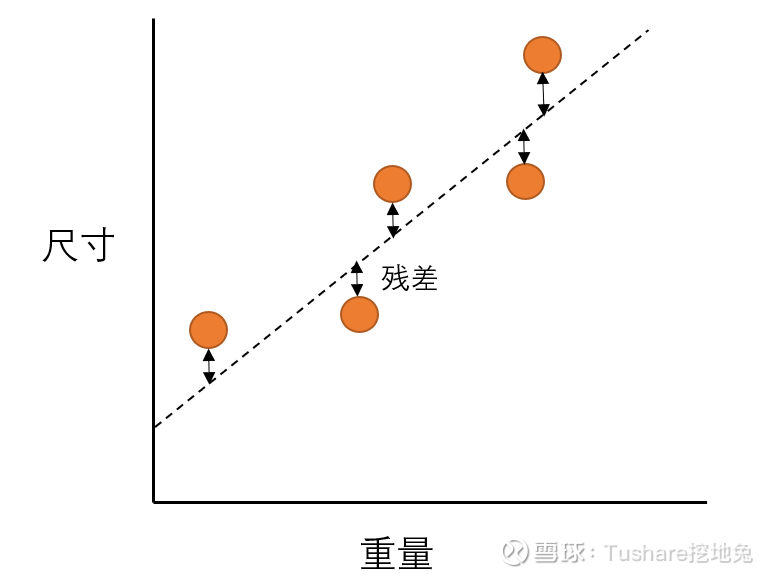③ 预测。① 获取数据，包含重量和它所属的类别，即是否肥胖。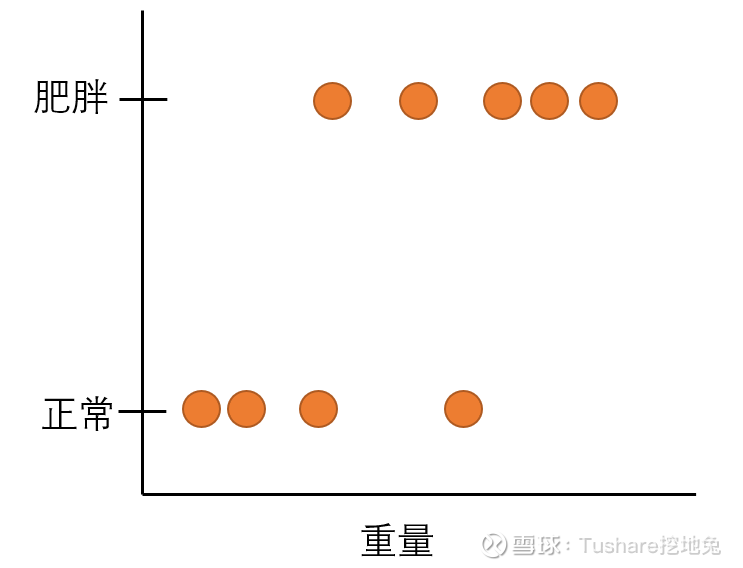② 建立模型，拟合出一条S曲线。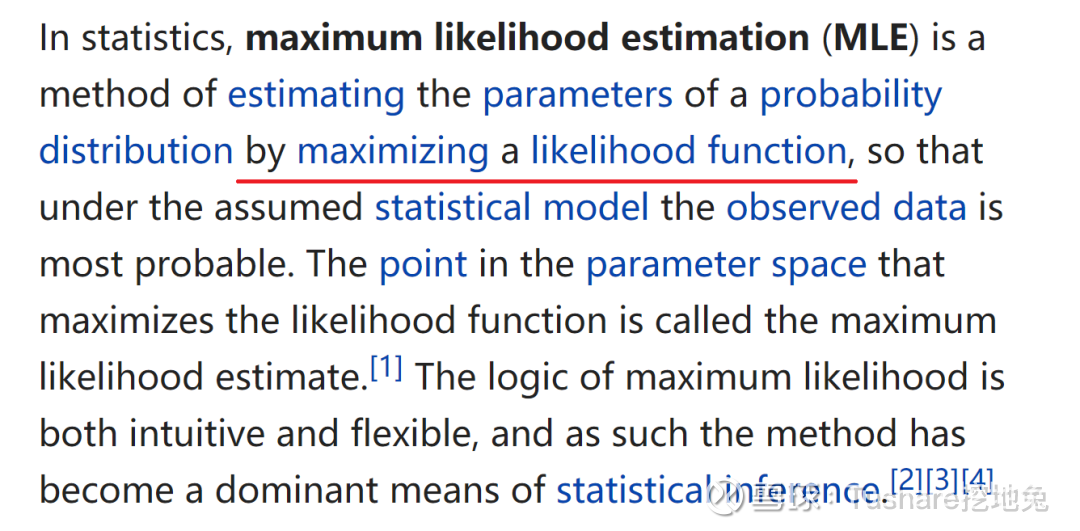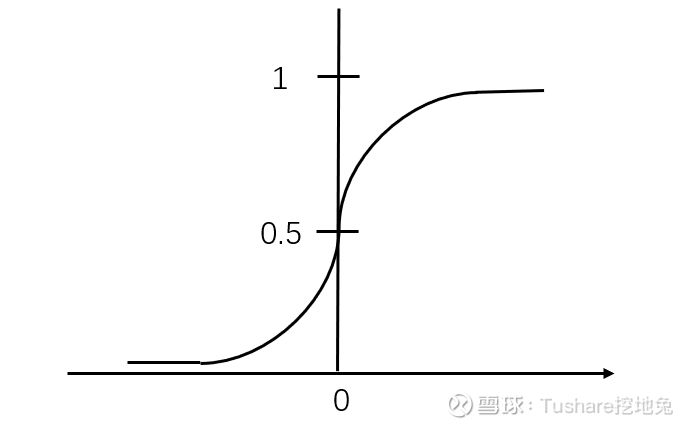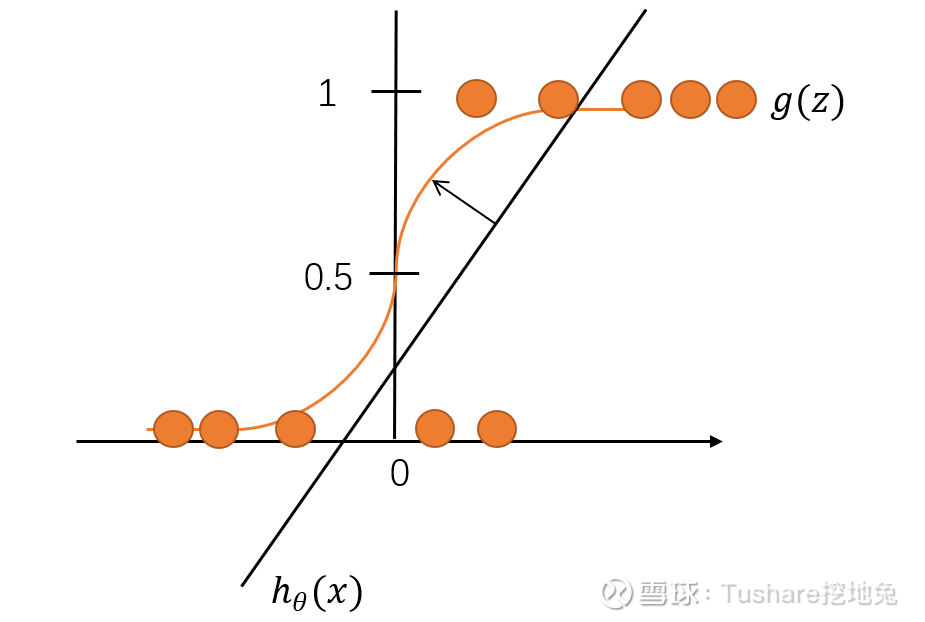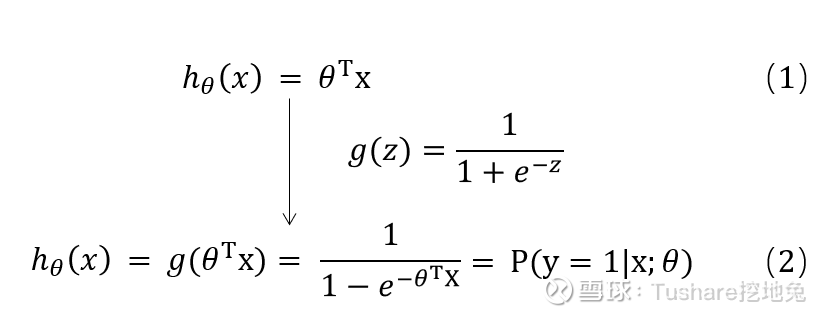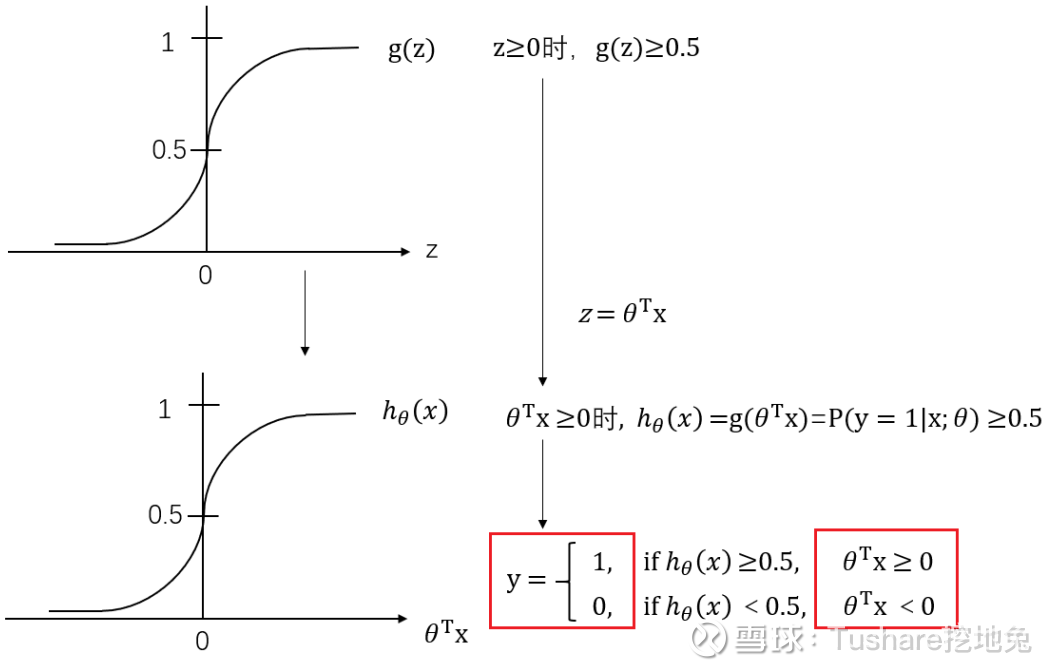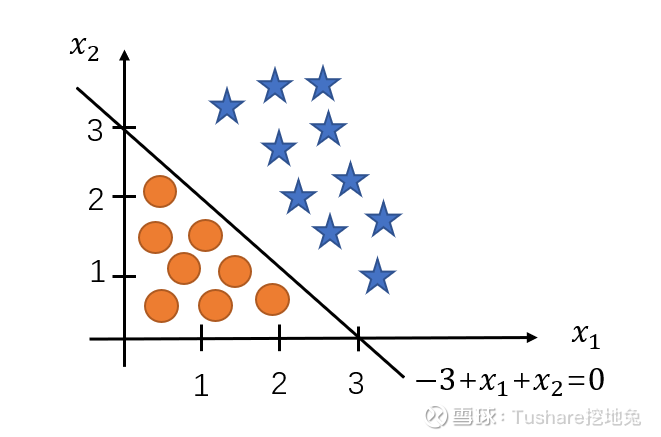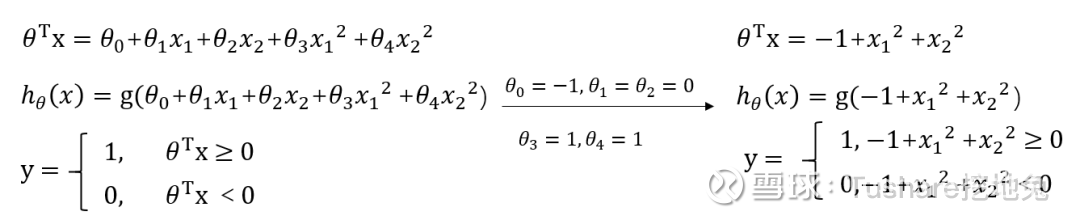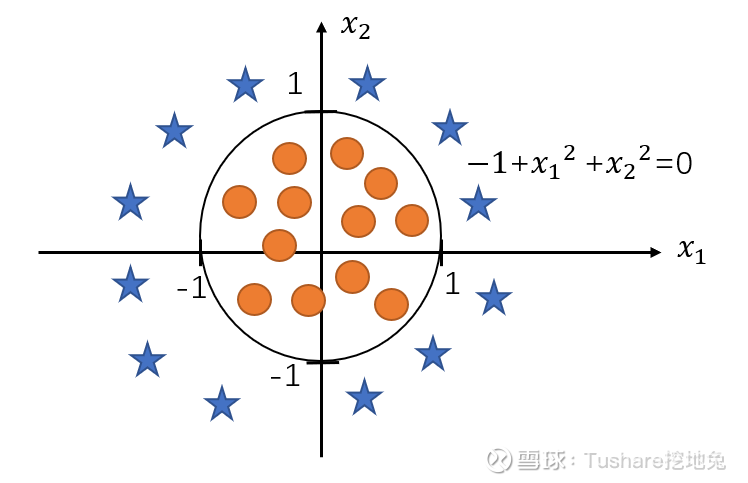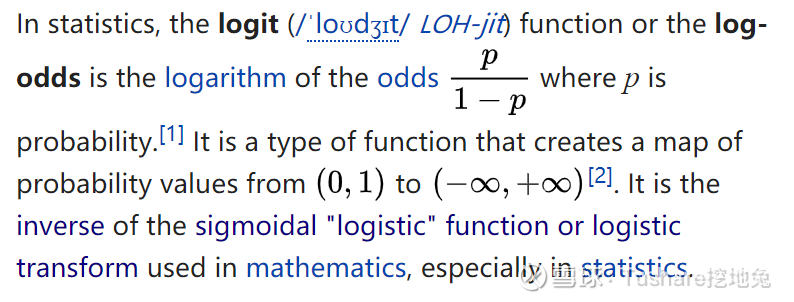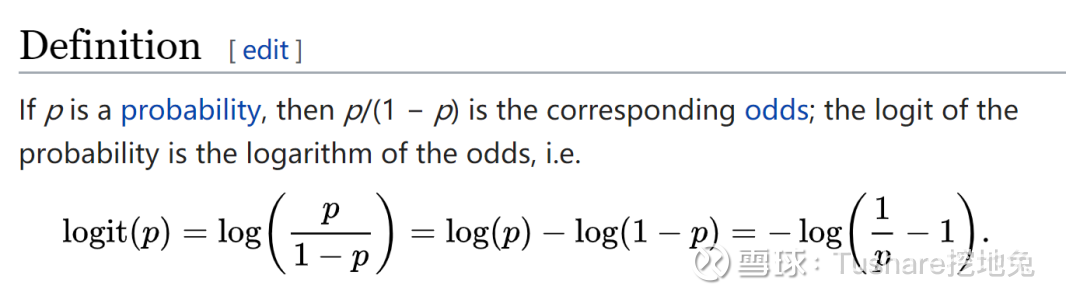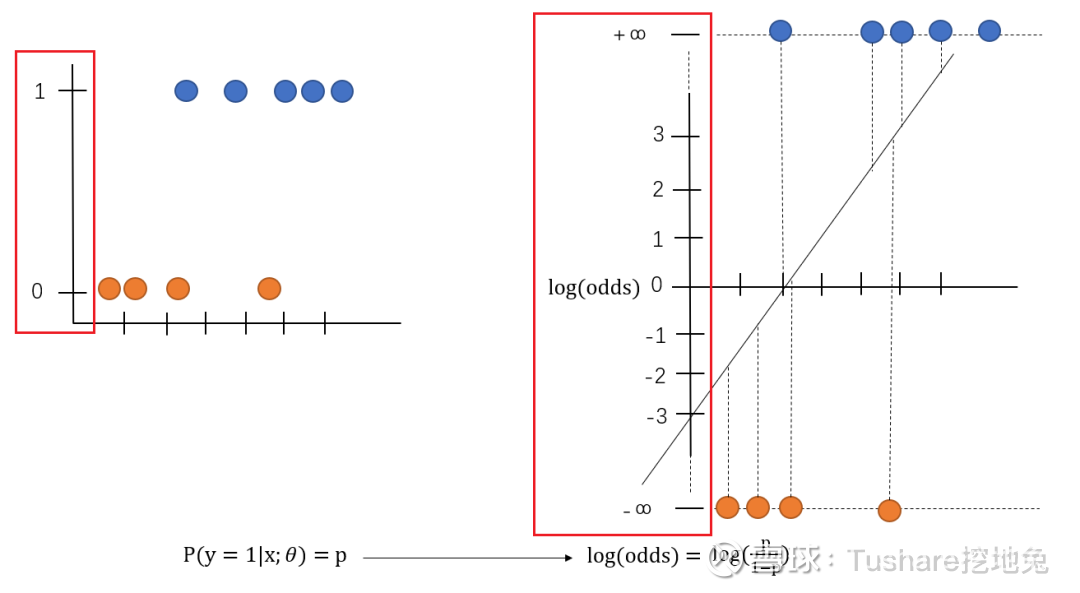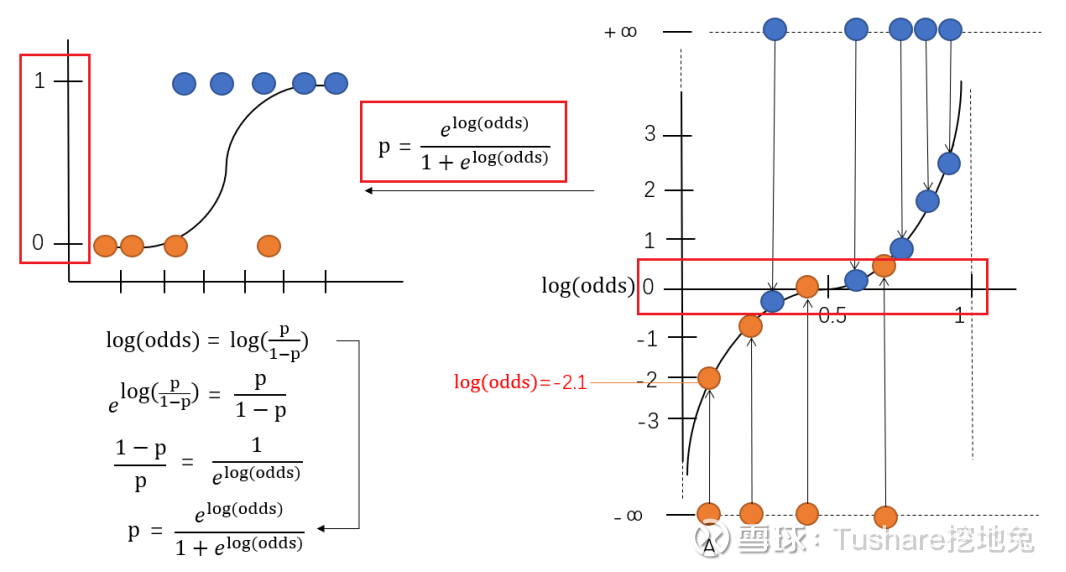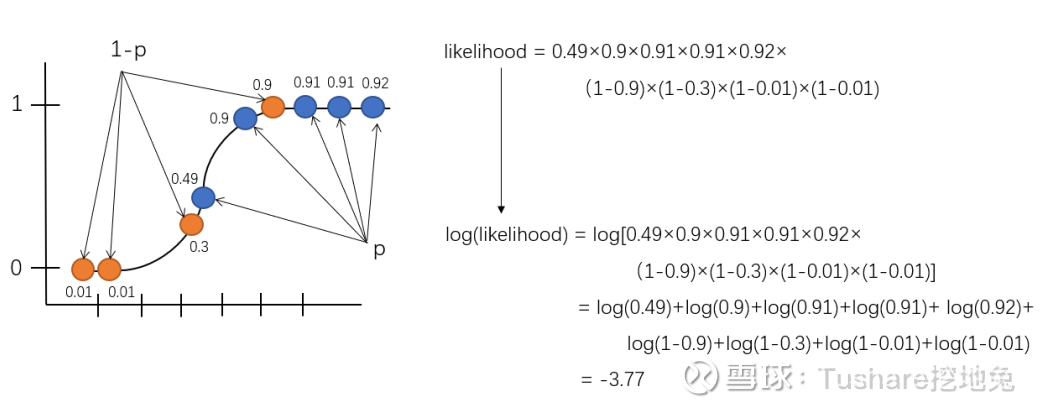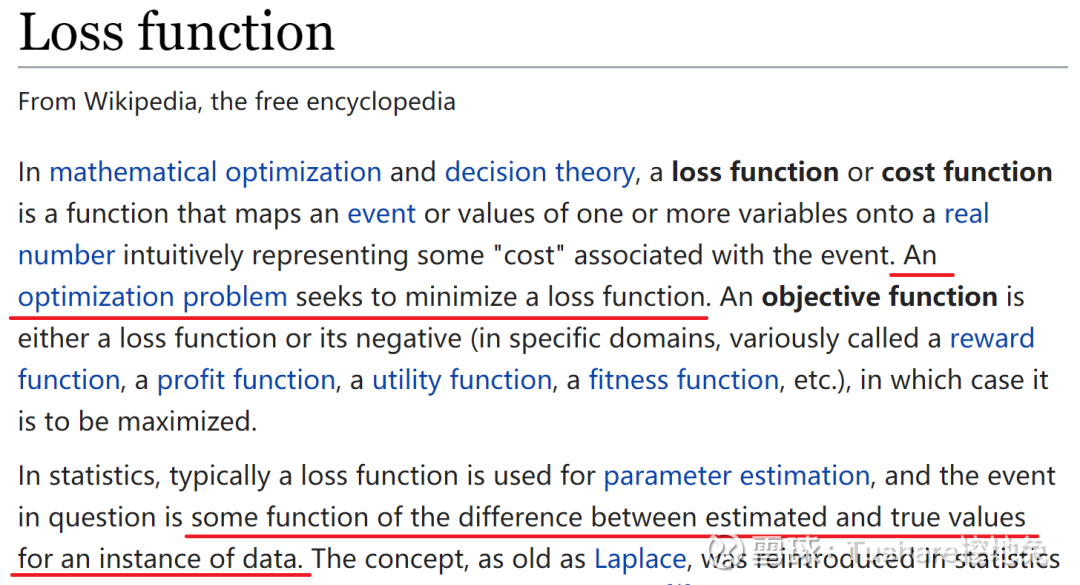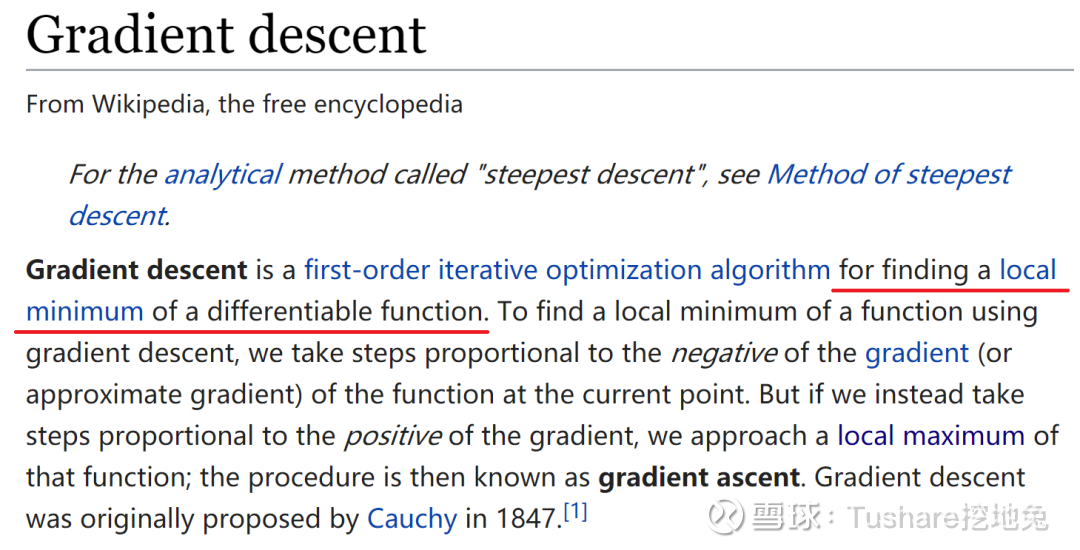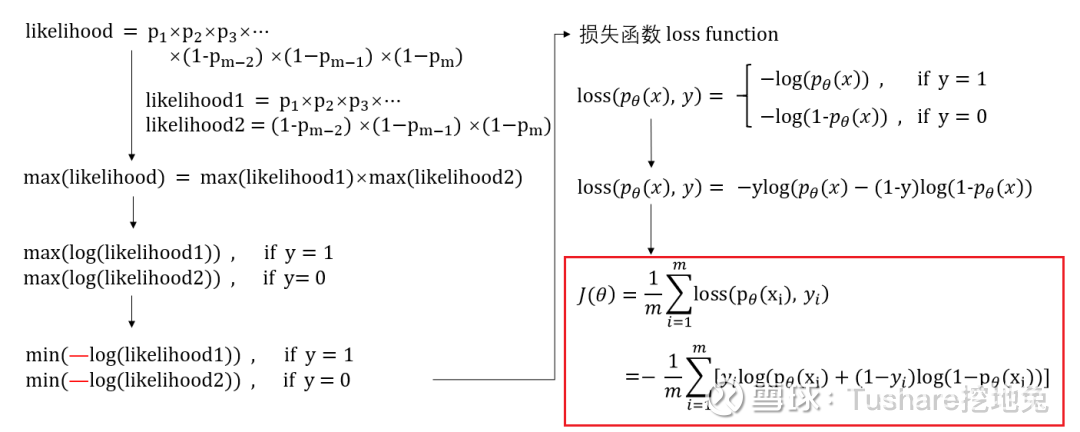Python 实例

① 先导入相应的模块

② 划分训练集和测试集

③ 创建一个分类器

④ 放入训练集数据进行学习，得到预测模型

⑤ 在测试集数据上进行预测

【工具】Python 3

【数据】tushare.pro

【注】本文注重的是方法的讲解，请大家灵活掌握。

import tushare as ts
import pandas as pd
pd.set_option("expand_frame_repr", False)       # 当列太多时不换行
# 设置token
pro = ts.pro_api()
# 导入000002.SZ前复权日线行情数据，保留收盘价列
df = ts.pro_bar(ts_code='000002.SZ', adj='qfq', start_date='20190101', end_date='20191231')
df = df[['close']]
# 计算当前、未来1-day涨跌幅
df['1d_future_close'] = df['close'].shift(-1)
df['1d_close_future_pct'] = df['1d_future_close'].pct_change(1)
df['1d_close_pct'] = df['close'].pct_change(1)
df.dropna(inplace=True)
# ====1代表上涨，0代表下跌
df.loc[df['1d_close_future_pct'] > 0, '未来1d涨跌幅方向'] = 1
df.loc[df['1d_close_future_pct'] df = df[['1d_close_pct', '未来1d涨跌幅方向']]
df.rename(columns={'1d_close_pct': '当前1d涨跌幅'}, inplace=True)
当前1d涨跌幅  未来1d涨跌幅方向
2019-01-03  0.007112        1.0
2019-01-04  0.035728        1.0
2019-01-07  0.004815        0.0
2019-01-08 -0.001997        1.0
2019-01-09  0.013203        0.0
from sklearn.linear_model import LogisticRegression
from sklearn.model_selection import train_test_split
# 创建特征 X 和标签 y
y = df['未来1d涨跌幅方向'].values
X = df.drop('未来1d涨跌幅方向', axis=1).values
# 划分训练集和测试集
X_train, X_test, y_train, y_test = train_test_split(X, y, test_size=0.4, random_state=42)
# 创建一个逻辑回归分类器
logreg = LogisticRegression(solver='liblinear')
# 放入训练集数据进行学习
logreg.fit(X_train, y_train)
# 在测试集数据上进行预测
new_prediction = logreg.predict(X_test)
print("Prediction: {}".format(new_prediction))
# 测算模型的表现：预测对的个数 / 总个数
print(logreg.score(X_test, y_test))
Prediction: [0. 0. 0. 0. 0. 0. 0. 0. 0. 0. 0. 0. 0. 0. 0. 0. 0. 0. 0. 0. 0. 0. 0. 0.
0. 0. 0. 0. 0. 0. 0. 0. 0. 0. 0. 0. 0. 0. 0. 0. 0. 0. 0. 0. 0. 0. 0. 0.
0. 0. 0. 0. 0. 0. 0. 0. 0. 0. 0. 0. 0. 0. 0. 0. 0. 0. 0. 0. 0. 0. 0. 0.
0. 0. 0. 0. 0. 0. 0. 0. 0. 0. 0. 0. 0. 0. 0. 0. 0. 0. 0. 0. 0. 0. 0. 0.
0.]0.4948453608247423

【推荐阅读】END

【参考链接】

Logistic Regression [Andrew Ng]【6】

Logistic Regression [StatQuest]【7】

 03-31 09:28 雪球 转发：0 回复：3 喜欢：3

## 全部评论

Tushare挖地兔04-06 09:23

ts.pro_bar(ts_code='600276.SH', adj='qfq', start_date='20100104', end_date='20100104')大佬，参数是2010年恒瑞医药的前复权价格，为啥得到的是不复权的价格呢？600276.SH 20100104 52.01